# Optimize Deep Learning GPU Operators with TVM: A Depthwise Convolution Example

Efficient deep learning operators are at the core of deep learning systems. Usually these operators are hard to optimize and require great efforts of HPC experts. TVM, an end to end tensor IR/DSL stack, makes this much easier.

This blog teaches you how to write high-performance GPU operator kernels with the help of TVM. We use depthwise convolution (i.e. topi.nn.depthwise_conv2d_nchw) as an example, and demonstrate how we can improve over the already hand optimized CUDA kernel in tensorflow. Our final version is 2x-4x faster than the optimized kernel in tf-1.2 under different workloads, and 3x-7x faster with operator fusion enabled. Below is the result tested on GTX1080, with filter size = [1, 256, 3, 3], stride = [1, 1], padding = ‘SAME’: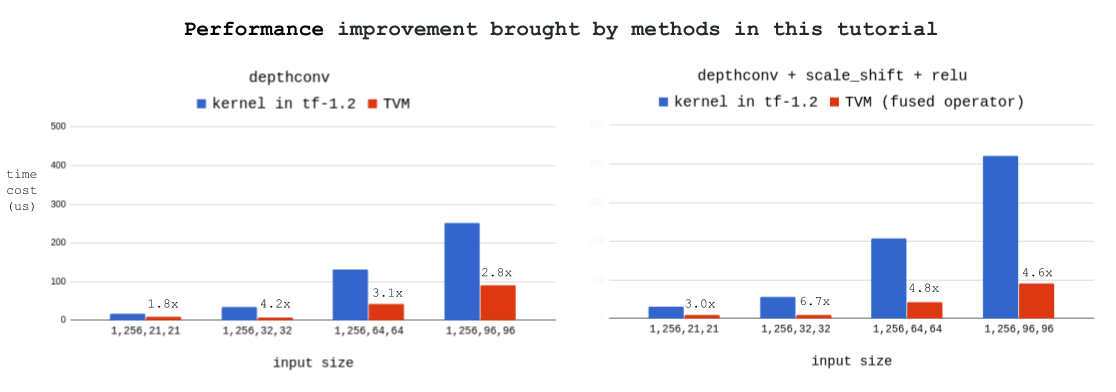## Introduction to Depthwise Convolution

Depthwise convolution is an important building block of modern architectures, such as Xception  and MobileNet . It’s an effective method to reduce the computation complexity of deep neural networks.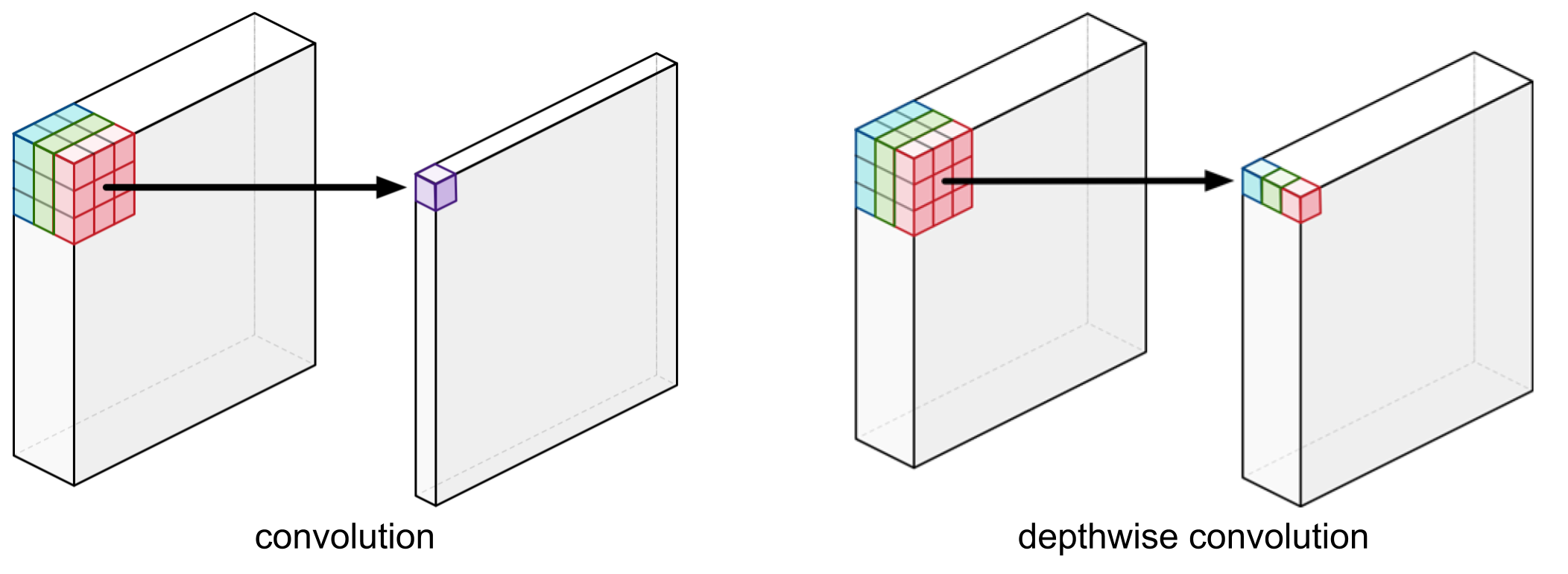In TVM, depthwise convolution can be declared as:

``````# padding stage
lambda b, c, i, j: tvm.select(
# depthconv stage
di = tvm.reduce_axis((0, filter_height), name='di')
dj = tvm.reduce_axis((0, filter_width), name='dj')
Output = tvm.compute(
(batch, out_channel, out_height, out_width),
lambda b, c, i, j: tvm.sum(
PaddedInput[b, c/channel_multiplier, i*stride_h + di, j*stride_w + dj] * Filter[c/channel_multiplier, c%channel_multiplier, di, dj],
axis=[di, dj]),
name='DepthwiseConv2d')
``````

## General GPU Optimization Guidelines

This part briefly talks about three concepts we should know when optimizing CUDA code: data reuse, shared memory and bank conflicts. It would be great if you already know them, then you may skip this part.

### Data Reuse

In modern computing architectures, the cost of loading data from memory is much higher than doing a single floating point computation . Because of that, we always want to reuse the input data after they are loaded into registers or shared memory (cache).

There are two forms of data reuse in depthwise convolution: filter reuse and input reuse. Filter reuse happens as the filter slides over the input channel and computes multiple times. Input reuse is realized through tiling, let’s take 3x3 depthwise conv as an example: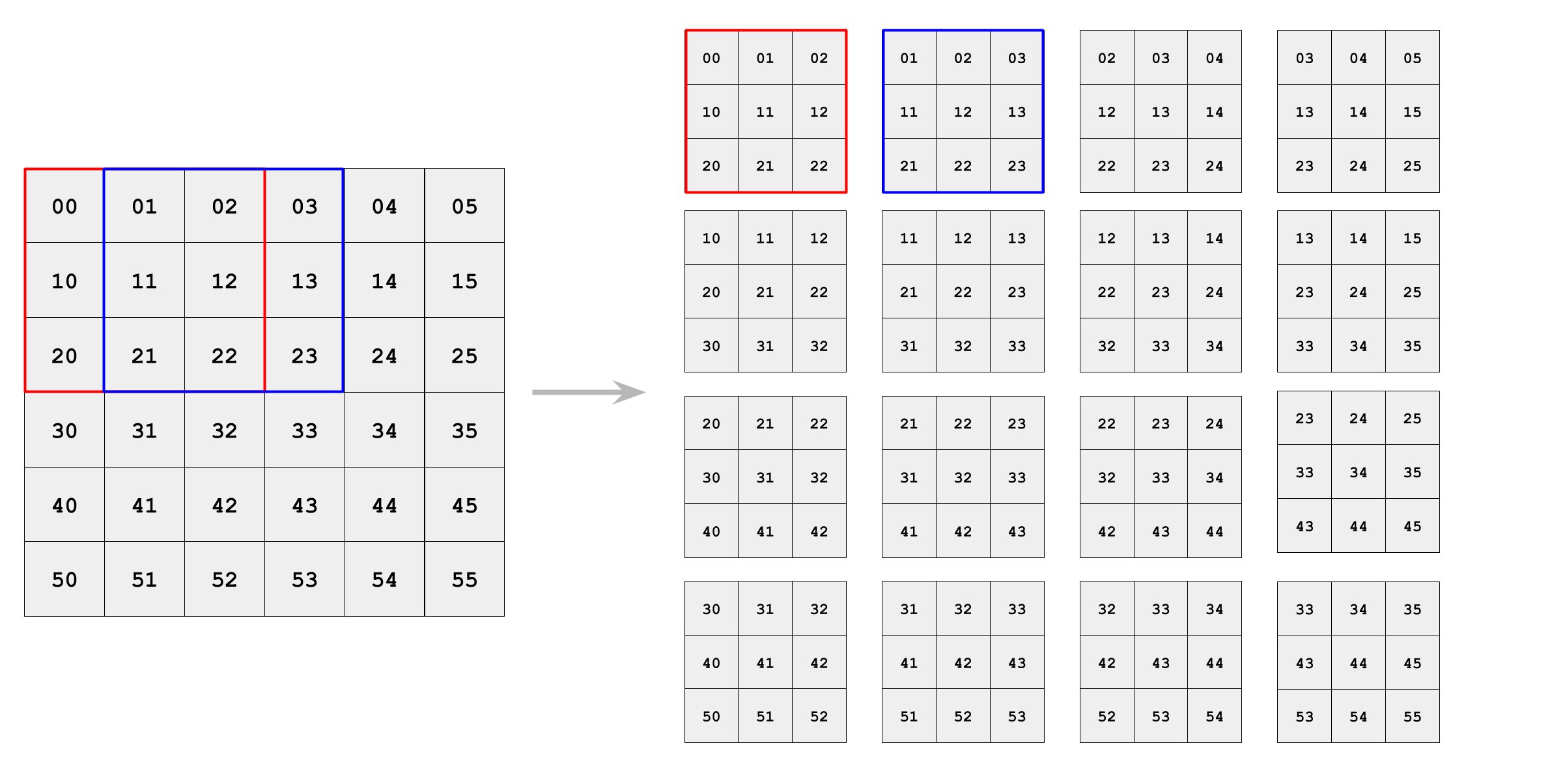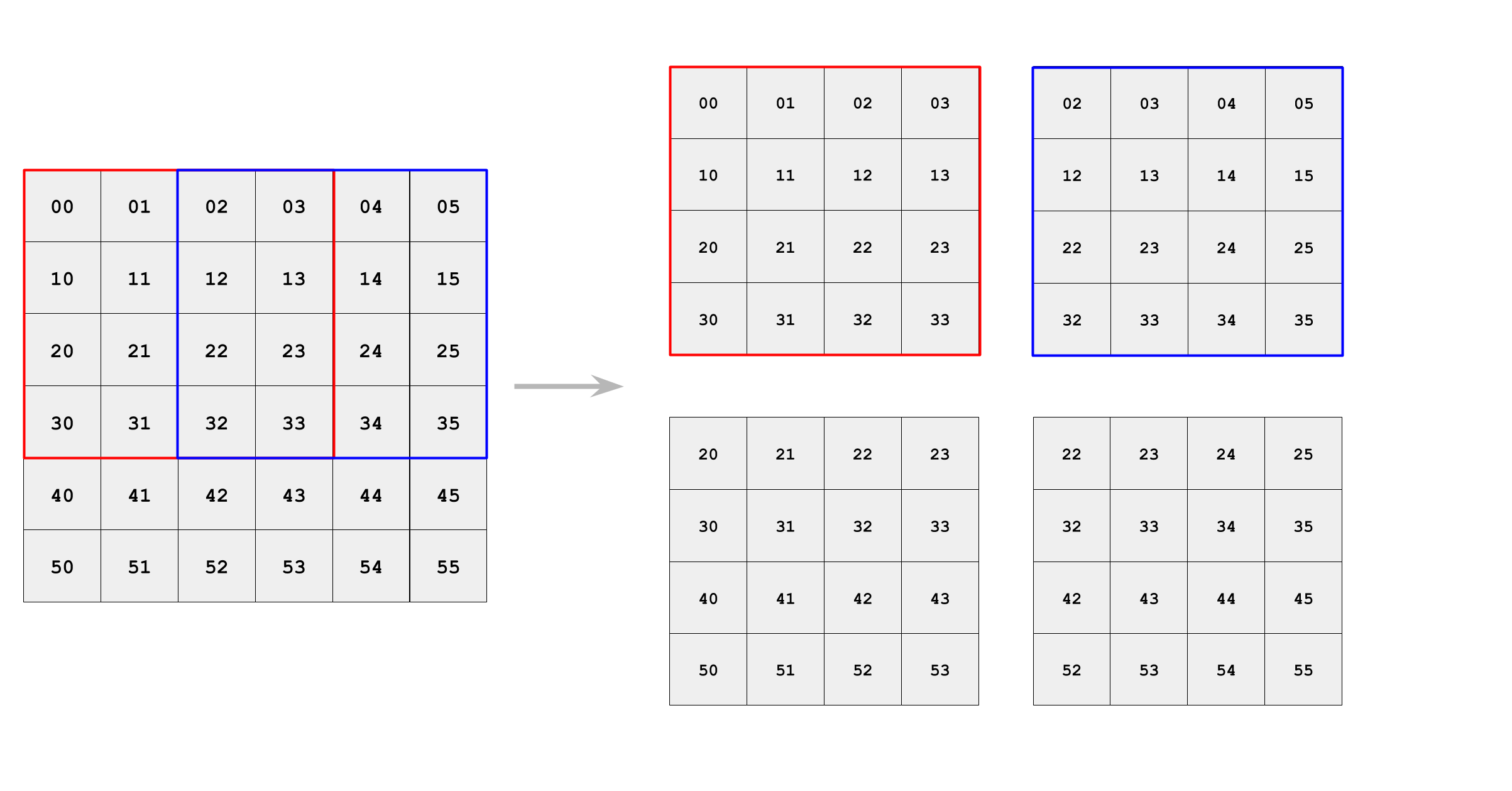### Shared Memory and Bank Conflicts

Shared memory can be seen as cache in GPU. It is on-chip and much faster than global memory.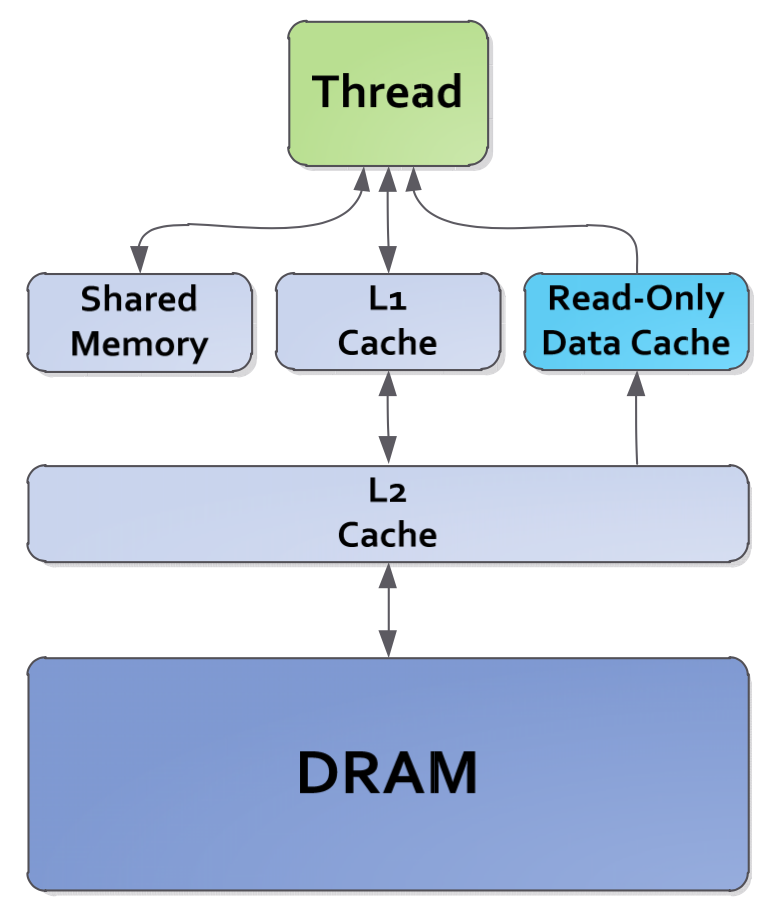Shared memory is allocated per block. It’s common practice to load data from global memory into shared memory, and then all threads in the block read data from shared memory.

The size of shared memory is limited (usually 48K), so we must be cautious of shared memory overflow. Besides, too much shared memory allocated to one block limits the number of active blocks per multiprocessor.

Another performance issue with shared memory is bank conflicts. Shared memory is divided into equally sized memory modules (banks) that can be accessed simultaneously, however, if multiple threads access the same memory bank (causing bank conflicts), the accesses will be serialized, thus decreasing the effective bandwidth.

Shared memory banks are organized such that successive addresses are assigned to successive banks. To avoid bank conflicts, it’s better that successive threads access successive memory addresses, as illustrated below (each color represents one shared memory bank):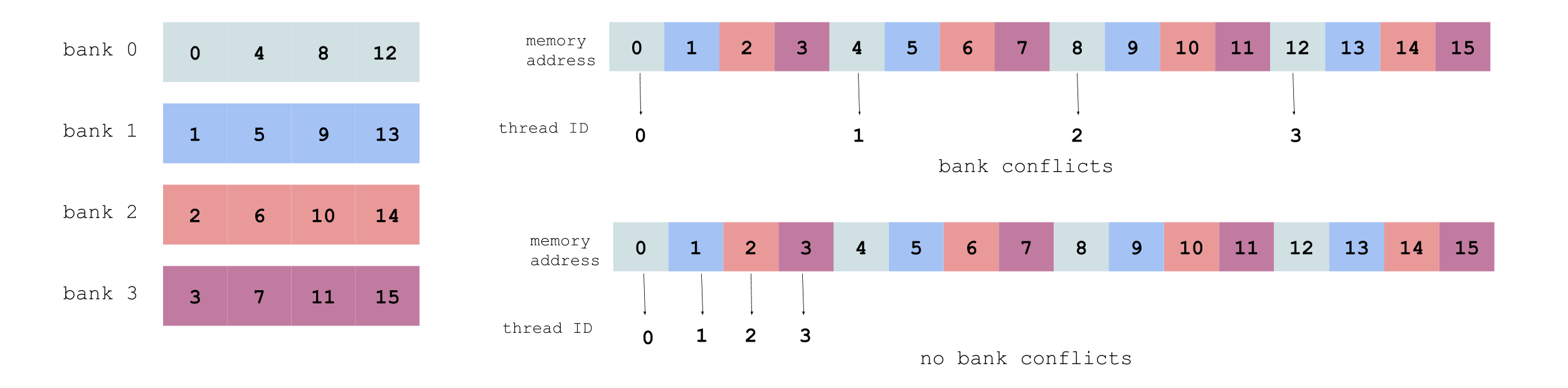For more details on shared memory and bank conflicts, please refer to this Nvidia’s blog.

Ok, now let’s start optimizing depthwise convolution in TVM.

## Schedule Optimization

### Compute PaddedInput Inline to Save Memory Allocation

As we see from part 1, padding is declared explicitly as a separate stage. We compute it inline to avoid redundant memory allocation:

``````s = tvm.create_schedule(Output.op)
``````

### Divide One Large Channel into Smaller Blocks

One straightforward schedule for depthwise convolution is that one cuda block takes care of one input channel and corresponding filters, loading them into shared memory and then computing:

``````IS = s.cache_read(PaddedInput, "shared", [DepthwiseConv2d])
# bind the dimension of batch (N in NCHW) with block_y
s[Output].bind(Output.op.axis, block_y)
# bind the dimension of channel (C in NCHW) with block_x
s[Output].bind(Output.op.axis, block_x)
``````

We test the average time cost of 1000 runs on GTX 1080, and compare with depthwise_conv2d in tensorflow. Here is the result:

[1, 256, 21, 21] [256, 1, 3, 3] [1, 1] 16.1 9.1
[1, 256, 32, 32] [256, 1, 3, 3] [1, 1] 34.8 14.5
[1, 256, 64, 64] [256, 1, 3, 3] [1, 1] 130.9 98.9
[1, 256, 96, 96] [256, 1, 3, 3] [1, 1] 251.6 387.4

As we can see, this schedule performs well with small channel size like 21 x 21 or 32 x 32, however, its performance drops seriously as the channel size increases to larger than 64 x 64. One main reason is that too much shared memory allocated to one block limits the number of active blocks per multiprocessor.

We modify the schedule to divide one large channel into smaller blocks. For example, one channel (64 x 64 or 96 x 96) is divided into blocks of 32 x 32, and one cuda block takes care of one 32 x 32 block:

``````blocking_h = 32
blocking_w = 32
# split the dimension of height (H in NCHW)
bx1, _ = s[Output].split(Output.op.axis, factor=blocking_h)
# split the dimension of width (W in NCHW)
bx2, _ = s[Output].split(Output.op.axis, factor=blocking_w)
# assign one 32 x 32 block to one cuda block
by = s[Output].fuse(Output.op.axis, Output.op.axis)
s[Output].bind(by, block_y)
bx = s[Output].fuse(bx1, bx2)
s[Output].bind(bx, block_x)
``````

Here is the new result:

[1, 256, 64, 64] [32, 32] 130.9 63.4
[1, 256, 96, 96] [32, 32] 251.6 132.5

Our blocking strategy works! For 64 x 64 channel size, it brings 1.6x acceleration (98.9us -> 63.4us); for 96 x 96 channel size, it brings 2.9x acceleration (387.4us -> 132.5us).

### Tuning Parameters of Thread Numbers

How to schedule the workload, say, 32x32 among the threads of one cuda block? Intuitively, it should be like this:

``````num_thread_y = 8
s[Output].reorder(ty, tx, yi, xi)
``````

There are two parameters in the schedule: `num_thread_y` and `num_thread_x`. How to determine the optimal combination of them? Well, let’s first do some experiments. Below is the result with Filter = [256, 1, 3, 3] and stride = [1, 1]:

1 [1, 256, 32, 32] 8 32 9.7
2 [1, 256, 32, 32] 4 32 8.8
3 [1, 256, 32, 32] 1 32 17.7
4 [1, 256, 32, 32] 32 1 32.5

Many interesting observations from above results:

• Case 2 is faster than case 1. In case 2, each thread computes a 8x1 tile in output, which corresponds to a 10x3 tile in input. It has better data reuse than case 1’s 4x1 tile.

• Case 3 is slower than case 2. It’s because in case 3, the workload per thread is too large and leads to much cost of local memory read.

• Case 4 is slower than case 3. It’s because `num_thread_x = 32` ensures no bank conflicts, while `num_thread_y = 32` doesn’t.

To summarize what we learn from above observations:

• Large tile is good for data reuse, but not good for local memory read.
• The influence of `num_thread_y` and `num_thread_x` on bank conflicts is asymmetric.
• To find the optimal combination of `num_thread_y` and `num_thread_x` is to achieve a balance of efficient shared memory access (avoid bank conflicts), data reuse, and local memory read.

Pretty tricky. So, what exactly should we do to find the optimal combination? The answer is brute force search. We can pass `num_thread_y` and `num_thread_x` as arguments to the schedule function, and try all possible combinations to find the optimal one. This can be done easily in TVM:

``````def schedule_depthwise_conv2d(..., num_thread_y=8, num_thread_x=8):
do_schedule_as_usual
return schedule

min_time_cost = inf
time_cost = test_depthwise_conv2d(..., schedule)
if time_cost < min_time_cost:
min_time_cost = time_cost
``````

In fact, it can be seen as a simple auto scheduler.

Vthread (virtual thread) in TVM is introduced to support strided patterns. We can use it this way:

``````num_vthread_y = 2
# split the dimension of height (H in NCHW) twice
# split the dimension of width (W in NCHW) twice
s[Output].reorder(tvy, tvx, ty, tx, yi, xi)
``````

Let’s print the IR to see what vthread does:

``````/* Input = [1, 1, 32, 32], Filter = [1, 1, 3, 3], stride = [1, 1], padding = 'SAME' */
produce DepthwiseConv2d {
// attr [iter_var(blockIdx.y, , blockIdx.y)] thread_extent = 1
// attr [iter_var(blockIdx.x, , blockIdx.x)] thread_extent = 1
for (i.inner.inner.inner, 0, 2) {
for (j.inner.inner.inner, 0, 2) {
DepthwiseConv2d[((((((((blockIdx.y + blockIdx.x)*16) + threadIdx.y)*32) + threadIdx.x)*2) + (i.inner.inner.inner*32)) + j.inner.inner.inner)] = 0.000000f
DepthwiseConv2d[(((((((((blockIdx.y + blockIdx.x)*16) + threadIdx.y)*32) + threadIdx.x)*2) + (i.inner.inner.inner*32)) + j.inner.inner.inner) + 512)] = 0.000000f
DepthwiseConv2d[(((((((((blockIdx.y + blockIdx.x)*16) + threadIdx.y)*32) + threadIdx.x)*2) + (i.inner.inner.inner*32)) + j.inner.inner.inner) + 16)] = 0.000000f
DepthwiseConv2d[(((((((((blockIdx.y + blockIdx.x)*16) + threadIdx.y)*32) + threadIdx.x)*2) + (i.inner.inner.inner*32)) + j.inner.inner.inner) + 528)] = 0.000000f
for (di, 0, 3) {
for (dj, 0, 3) {
DepthwiseConv2d[((((((((blockIdx.y + blockIdx.x)*16) + threadIdx.y)*32) + threadIdx.x)*2) + (i.inner.inner.inner*32)) + j.inner.inner.inner)] = (DepthwiseConv2d[((((((((blockIdx.y + blockIdx.x)*16) + threadIdx.y)*32) + threadIdx.x)*2) + (i.inner.inner.inner*32)) + j.inner.inner.inner)] + (tvm_if_then_else(((((((1 - di) - i.inner.inner.inner) <= (((blockIdx.x*16) + threadIdx.y)*2)) && ((((blockIdx.x*16) + threadIdx.y)*2) < ((33 - di) - i.inner.inner.inner))) && (((1 - dj) - j.inner.inner.inner) <= (threadIdx.x*2))) && ((threadIdx.x*2) < ((33 - dj) - j.inner.inner.inner))), Input[(((((((((((blockIdx.y + blockIdx.x)*16) + threadIdx.y)*32) + threadIdx.x)*2) + (i.inner.inner.inner*32)) + j.inner.inner.inner) + (di*32)) + dj) + -33)], 0.000000f)*Filter[((di*3) + dj)]))
DepthwiseConv2d[(((((((((blockIdx.y + blockIdx.x)*16) + threadIdx.y)*32) + threadIdx.x)*2) + (i.inner.inner.inner*32)) + j.inner.inner.inner) + 512)] = (DepthwiseConv2d[(((((((((blockIdx.y + blockIdx.x)*16) + threadIdx.y)*32) + threadIdx.x)*2) + (i.inner.inner.inner*32)) + j.inner.inner.inner) + 512)] + (tvm_if_then_else(((((((-15 - di) - i.inner.inner.inner) <= (((blockIdx.x*16) + threadIdx.y)*2)) && ((((blockIdx.x*16) + threadIdx.y)*2) < ((17 - di) - i.inner.inner.inner))) && (((1 - dj) - j.inner.inner.inner) <= (threadIdx.x*2))) && ((threadIdx.x*2) < ((33 - dj) - j.inner.inner.inner))), Input[(((((((((((blockIdx.y + blockIdx.x)*16) + threadIdx.y)*32) + threadIdx.x)*2) + (i.inner.inner.inner*32)) + j.inner.inner.inner) + (di*32)) + dj) + 479)], 0.000000f)*Filter[((di*3) + dj)]))
DepthwiseConv2d[(((((((((blockIdx.y + blockIdx.x)*16) + threadIdx.y)*32) + threadIdx.x)*2) + (i.inner.inner.inner*32)) + j.inner.inner.inner) + 16)] = (DepthwiseConv2d[(((((((((blockIdx.y + blockIdx.x)*16) + threadIdx.y)*32) + threadIdx.x)*2) + (i.inner.inner.inner*32)) + j.inner.inner.inner) + 16)] + (tvm_if_then_else(((((((1 - di) - i.inner.inner.inner) <= (((blockIdx.x*16) + threadIdx.y)*2)) && ((((blockIdx.x*16) + threadIdx.y)*2) < ((33 - di) - i.inner.inner.inner))) && (((-15 - dj) - j.inner.inner.inner) <= (threadIdx.x*2))) && ((threadIdx.x*2) < ((17 - dj) - j.inner.inner.inner))), Input[(((((((((((blockIdx.y + blockIdx.x)*16) + threadIdx.y)*32) + threadIdx.x)*2) + (i.inner.inner.inner*32)) + j.inner.inner.inner) + (di*32)) + dj) + -17)], 0.000000f)*Filter[((di*3) + dj)]))
DepthwiseConv2d[(((((((((blockIdx.y + blockIdx.x)*16) + threadIdx.y)*32) + threadIdx.x)*2) + (i.inner.inner.inner*32)) + j.inner.inner.inner) + 528)] = (DepthwiseConv2d[(((((((((blockIdx.y + blockIdx.x)*16) + threadIdx.y)*32) + threadIdx.x)*2) + (i.inner.inner.inner*32)) + j.inner.inner.inner) + 528)] + (tvm_if_then_else(((((((-15 - di) - i.inner.inner.inner) <= (((blockIdx.x*16) + threadIdx.y)*2)) && ((((blockIdx.x*16) + threadIdx.y)*2) < ((17 - di) - i.inner.inner.inner))) && (((-15 - dj) - j.inner.inner.inner) <= (threadIdx.x*2))) && ((threadIdx.x*2) < ((17 - dj) - j.inner.inner.inner))), Input[(((((((((((blockIdx.y + blockIdx.x)*16) + threadIdx.y)*32) + threadIdx.x)*2) + (i.inner.inner.inner*32)) + j.inner.inner.inner) + (di*32)) + dj) + 495)], 0.000000f)*Filter[((di*3) + dj)]))
}
}
}
}
}
``````

Without vthread (just set to 1), the IR is:

``````/* Input = [1, 1, 32, 32], Filter = [1, 1, 3, 3], stride = [1, 1], padding = 'SAME' */
produce DepthwiseConv2d {
// attr [iter_var(blockIdx.y, , blockIdx.y)] thread_extent = 1
// attr [iter_var(blockIdx.x, , blockIdx.x)] thread_extent = 1
for (i.inner.inner.inner, 0, 4) {
for (j.inner.inner.inner, 0, 4) {
DepthwiseConv2d[((((((((blockIdx.y + blockIdx.x)*8) + threadIdx.y)*32) + threadIdx.x)*4) + (i.inner.inner.inner*32)) + j.inner.inner.inner)] = 0.000000f
for (di, 0, 3) {
for (dj, 0, 3) {
DepthwiseConv2d[((((((((blockIdx.y + blockIdx.x)*8) + threadIdx.y)*32) + threadIdx.x)*4) + (i.inner.inner.inner*32)) + j.inner.inner.inner)] = (DepthwiseConv2d[((((((((blockIdx.y + blockIdx.x)*8) + threadIdx.y)*32) + threadIdx.x)*4) + (i.inner.inner.inner*32)) + j.inner.inner.inner)] + (tvm_if_then_else(((((((1 - di) - i.inner.inner.inner) <= (((blockIdx.x*8) + threadIdx.y)*4)) && ((((blockIdx.x*8) + threadIdx.y)*4) < ((33 - di) - i.inner.inner.inner))) && (((1 - dj) - j.inner.inner.inner) <= (threadIdx.x*4))) && ((threadIdx.x*4) < ((33 - dj) - j.inner.inner.inner))), Input[(((((((((((blockIdx.y + blockIdx.x)*8) + threadIdx.y)*32) + threadIdx.x)*4) + (i.inner.inner.inner*32)) + j.inner.inner.inner) + (di*32)) + dj) + -33)], 0.000000f)*Filter[((di*3) + dj)]))
}
}
}
}
}
``````

As we can see, when `num_vthread_y = 2` and `num_vthread_x = 2`, the 32 x 32 channel is divided into four sub-channels of 16 x 16. Each thread computes four output elements at a time, one element in one sub-channel.

Below is the result with Filter = [256, 1, 3, 3], stride = [1, 1], blocking_h = 32, blocking_w = 32:

1 [1, 256, 96, 96] 8, 8 1, 1 132.5
2 [1, 256, 96, 96] 8, 8 1, 4 103.1
3 [1, 256, 96, 96] 4, 32 1, 1 95.9
4 [1, 256, 96, 96] 8, 16 1, 2 90.9

Case 2 is faster than case 1. It’s because in case 2 `num_thread_x=8` and `num_vthread_x=4` together ensures that consecutive threads access consecutive memory addresses, thus avoiding bank conflicts, as illustrated below (each color represents one thread’s workload):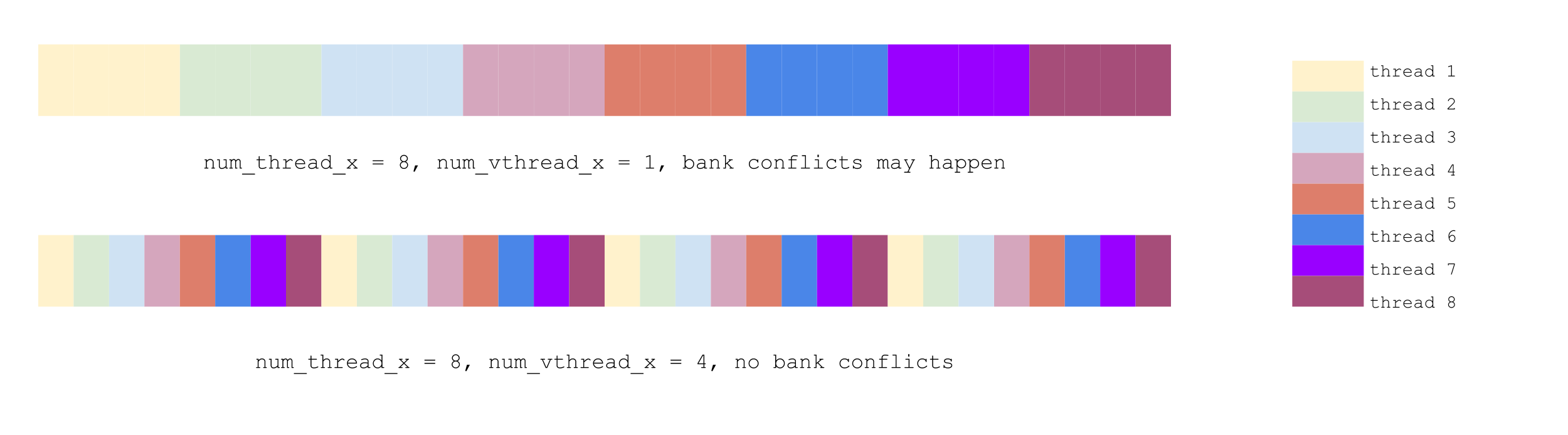In theory case 3 and 4 should be the same fast, since they have the same workload per thread, and both enjoy efficient shared memory access. Somehow case 4 is just a little faster.

Still remember tensorflow’s speed? It’s 251.6us, and now TVM is 2.8x faster. 387.4 -> 132.5 -> 95.9 -> 90.9, blocking helps the most; tuning thread numbers saves 37us; vthread saves additional 5us.

In fact, TVM can be extremely faster than tensorflow with large kernel size or channel_multiplier (because of more filter reuse) :

Input Filter stride tf-1.2 SAME pad (us) TVM SAME pad (us) How faster is TVM
[1, 256, 96, 96] [256, 1, 3, 3] [1, 1] 251.6 90.9 2.8x
[1, 256, 96, 96] [256, 1, 5, 5] [1, 1] 597.6 128.9 4.6x
[1, 256, 96, 96] [256, 2, 3, 3] [1, 1] 659.9 143.7 4.6x
[1, 256, 96, 96] [256, 2, 5, 5] [1, 1] 1203.9 170.5 7.1x

## Operator Fusion

One typical optimization we can do in deep learning is operator fusion, that computes multiple operators together in a single kernel without saving intermediate results back to global memory. TVM supports that out of the box.

Consider a common pattern in neural networks: `depthwise_conv2d` + `scale_shift` + `relu`. We can fuse the three operators into one, by slightly modifying the original schedule:

``````DepthwiseConv2d = topi.nn.depthwise_conv2d(Input, Filter, stride, padding)
ScaleShift = topi.nn.scale_shift(DepthwiseConv2d, Scale, Shift)
Relu = topi.nn.relu(ScaleShift)

Output = Relu # is no longer DepthwiseConv2d
s[ScaleShift].compute_inline() # this line fuses ScaleShift, explicitly
s[DepthwiseConv2d].set_scope("local") # this line fuses DepthwiseConv2d, implicitly
schedule(Output) # schedule for Output the same way we schedule for DepthwiseConv2d as discussed above
s[DepthwiseConv2d].compute_at(s[Output], tx) # tx is the inner most axis, bound to threadIdx.x
``````

It generates IR like this:

``````/* Input = [1, 1, 32, 32], Filter = [1, 1, 3, 3], stride = [1, 1], padding = 'SAME' */
produce Relu {
// attr [iter_var(blockIdx.y, , blockIdx.y)] thread_extent = 1
// attr [DepthwiseConv2d] storage_scope = "local"
allocate DepthwiseConv2d[float32 * 1 * 1 * 4 * 4]
// attr [iter_var(blockIdx.x, , blockIdx.x)] thread_extent = 1
produce DepthwiseConv2d {
for (i, 0, 4) {
for (j, 0, 4) {
DepthwiseConv2d[((i*4) + j)] = 0.000000f
for (di, 0, 3) {
for (dj, 0, 3) {
DepthwiseConv2d[((i*4) + j)] = (DepthwiseConv2d[((i*4) + j)] + (tvm_if_then_else(((((((1 - di) - i) <= (((blockIdx.x*8) + threadIdx.y)*4)) && ((((blockIdx.x*8) + threadIdx.y)*4) < ((33 - di) - i))) && (((1 - dj) - j) <= (threadIdx.x*4))) && ((threadIdx.x*4) < ((33 - dj) - j))), Input[(((((((((((blockIdx.y + blockIdx.x)*8) + threadIdx.y)*32) + threadIdx.x)*4) + (i*32)) + j) + (di*32)) + dj) + -33)], 0.000000f)*Filter[((di*3) + dj)]))
}
}
}
}
}
for (i2.inner.inner.inner, 0, 4) {
for (i3.inner.inner.inner, 0, 4) {
Relu[((((((((blockIdx.y + blockIdx.x)*8) + threadIdx.y)*32) + threadIdx.x)*4) + (i2.inner.inner.inner*32)) + i3.inner.inner.inner)] = max(((DepthwiseConv2d[((i2.inner.inner.inner*4) + i3.inner.inner.inner)]*Scale) + Shift), 0.000000f)
}
}
}
``````

As we can see, each thread computes `scale_shift` and `relu` before writing the result of `depthwise_conv2d` to global memory. The fused operator is as fast as single `depthwise_conv2d`. Below is the result with Input = [1, 256, 96, 96], Filter = [256, 1, 3, 3], stride = [1, 1], padding = ‘SAME’:

• tf-1.2 `depthwise_conv2d`: 251.6 us
• tf-1.2 `depthwise_conv2d` + `scale_shift` + `relu` (separate): 419.9 us
• TVM `depthwise_conv2d`: 90.9 us
• TVM `depthwise_conv2d + scale_shift + relu` (fused): 91.5 us

The advantage of operator fusion is obvious.

This is not the end, TVM can do operator fusion in a smarter way. You may refer to this and read the source code provided below.

## Acknowledgements

The author has many thanks to Tianqi Chen for his helpful advice and inspiring discussion.

## Bio

Yuwei Hu is an intern in Tusimple’s HPC group. He is experiencing a gap year after obtaining a bachelor’s degree in electrical engineering from Beihang University.

• Copyright © 2020 The Apache Software Foundation. Apache TVM, Apache, the Apache feather, and the Apache TVM project logo are either trademarks or registered trademarks of the Apache Software Foundation.